# Apply a function to an integration mapping

Who can use this feature?

Use the Function Editor to enhance data integrations using formulas within the mappings (e.g., math, text, date, logical, formatting).

The Function Editor is available for the following connectors and integrations:

1. Inbound: All connectors
2. Outbound: Salesforce and Hubspot connections

See all supported formulas. See source field formatting for additional parameters. A common use case for account and user integrations is to add a constant function. To see examples of this use case, check out this video.

## Add a function to an integration

1. Create a new integration or edit an existing integration job within Customer Data Hub.
2. In the mapping table, hover over the source field that you want to edit or add a new field (e.g., adding a constant value), and click Open Function Editor.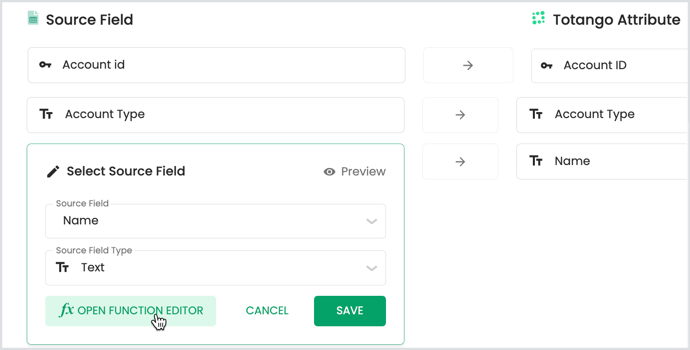3. In the Function Editor, click Add Function. Choose from math, logical, text, date, or formatting.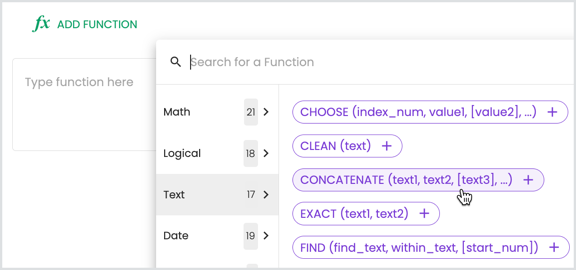4. For each field that you want to add to the function, click Add as parameter.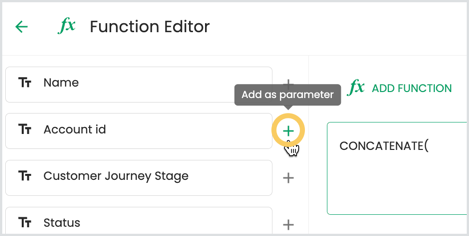5. When done editing the function, click Preview.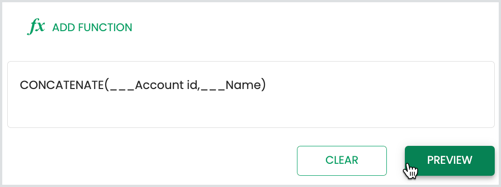6. If the preview looks as expected, click Save Function.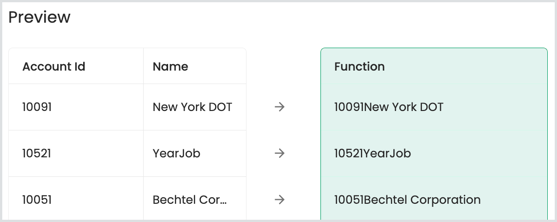The function is added to the mapping.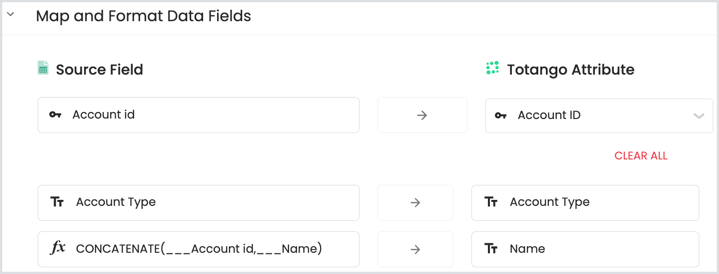7. Click Validate Mapping and save the integration.

## Known limitations

• Using a formula field as part of another new formula is not supported.
• The maximum number of functions an integration job can have is 15.
• Formula parameters and arguments are limited to 510 characters long (except for LEFT function that can get a longer first parameter if it's used alone and not as part of another formula). See details.
• Formula fields are not supported for write-back to external data source.
• Formulas cannot be used for field names that have the characters: ,  ) = < >
• Find function returns an error when no occurrence is found.
• When importing data from CSV file with formulas mapping, validation is limited to first 1,000 rows.
• To make logical comparisons of dates use DATEVALUE. Example:  IF((DATEVALUE(___SomeDate)+30) < DATEVALUE(TODAY()),"P", "C").
• Use the VALUE() function to convert string values to numeric values.

## Convert values from scientific notation

When integrating a large number of fields into Totango, the values may appear in scientific notation.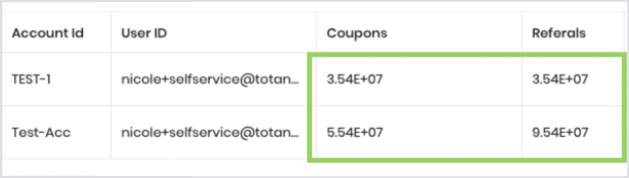Totango will not calculate the values correctly if used in a custom metric or other automatic calculation process. Use Function Editor to reformat values in scientific notation.

1. Within the integration job mapping, leave the values as a text field type and open the Function Editor.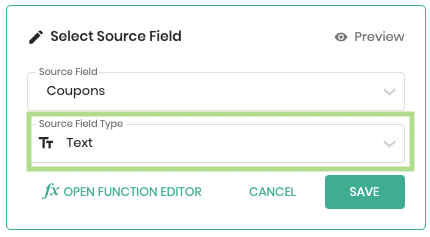2. Use the "VALUE" function to convert the number value from scientific notation to a number format like this: VALUE(<Field In Scientific Notation>). (Click the "+" button next to the field you want to convert from the left-hand panel.)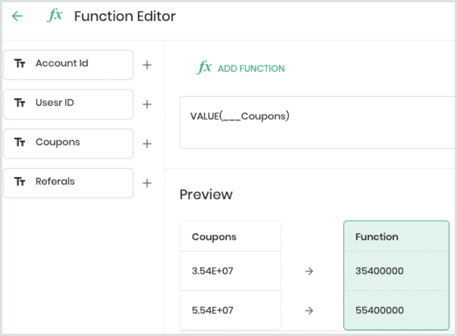If you would like to turn the value from scientific notation to currency you can use a formula like this: VALUE(TEXT(VALUE(<Field In Scientific Notation>), "\$0.00"))

3. Preview and save.
4. After adding applying the VALUE function to each field you want to convert, click Validate Mapping and save the integration.

The VALUE function converts text that appears in a recognized format (i.e. a number, date, or time format) into a numeric value. You must ensure the source field is a Text field type, or else the formula will not work (e.g., the "E" from the notation will be omitted).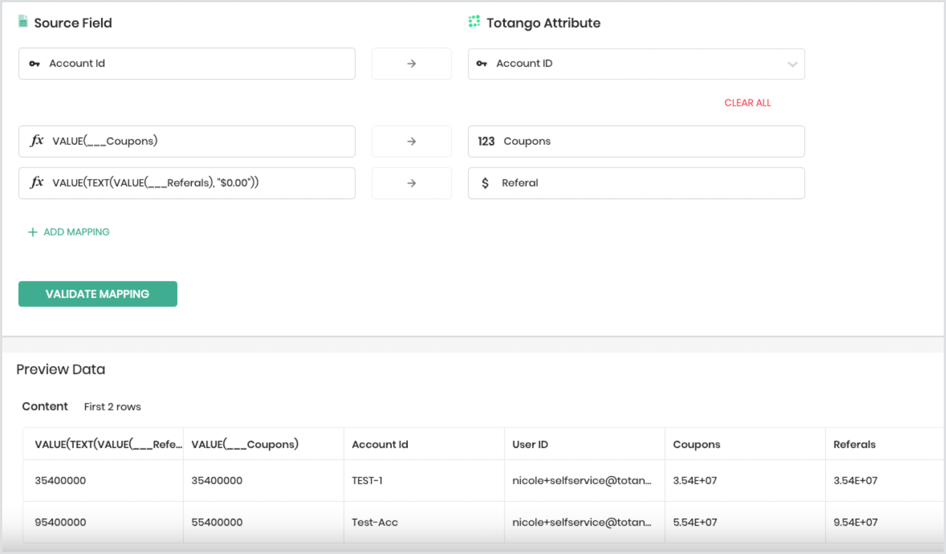## FAQs

Question: How do I calculate the number of days between two dates?

Answer: Refer to the supported formulas for details. For example, using a "CreateDate" attribute, we can construct a function to calculate the number of days between two dates, based on a 360 day year. Divide that number by 30 and round down to get the number of months since the date.

`ROUNDDOWN(DIVIDE(DAYS360(left(___CreatedDate, 10), TODAY()), 30), 0)`